If you have a related question, please click the "Ask a related question" button in the top right corner. The newly created question will be automatically linked to this question.

# AFE4404: AFE4404 Calibration code initCalibrationRoutine()

Part Number: AFE4404

Hi.

I was looking at the code Calibration_AFE4404.c initCalibrationRoutine() and left a question.

Can you tell me why the formula below came out?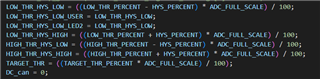Why do you save it in four variables using hysteresis percent: LOW_THR_HYS_LOW, LOW_THR_HYS_HIGH & HIGH_THR_HYS_LOW, HIGH_THR_HYS_HIGH?

• Hi,

App Engineer is OOO. Shall get back to you on Monday.

• Hi,

we will need more time to investigate this, hope to get back to you on 3/8.

• Hi,

The high and low thresholds are set in hysteresis percent to avoid frequent invoking of the calibration routine when the DC codes are closer to the threshold values.

i.e. it gives the DC codes some buffer zone/range/interval as long as the DC codes still within the tolerance range/interval so not to keep calibrating.

Thanks

Additionally, can I know why calibration_mode starts with s Ambient DAC instead of sInitialize?

If you look at the picture, you declared CALIBRATION_MODES calibration_mode = s AmbientDAC; with global variable.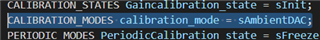Thank you!

• Hi,

I will get back to you around 3/10.

Thanks

• Hi,

The calibration mode begins with ambientDAC because the initCalibrationRoutine is called from Main when the user invokes calibration from GUI.

In the initCalibrationRoutine, the calibration mode is set to ambientDAC.

-------------------------------------------------

The documentation for the calibration routine is available in the documentation folder of the AFE4404 firmware source code available at https://www.ti.com/tool/AFE4404EVM#tech-docs

-------------------------

Thanks

• Hi,

I don't understand the formula below, so I leave a question.

I don't know why they split 65000.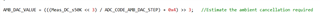Thank you.

• Hi,

What do you mean by split 65000?

Thanks

• What do you mean by split 65000?

If you look at the picture, you can see the formula AMB_DAC_VALUE = ((Meas_DC_s50K <<3) / ADC_CODE_AMB_DAC_STEP) + 0x4)> 3;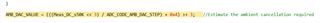Thank you

• Hi,

I will need some time to get back to you after internal discussion.

Thanks

• ADC_CODE_AMB_DAC_STEP_IDEAL is set to 87381 in the source code.

This variable corresponds to the ADC code for 50k gain and 0.5uA Offset DAC current.

(2*50e3*0.5e-6)*2^21/1.2

= 87381.33333333333333333333

ADC_CODE_AMB_DAC_STEP is set to 65000 to account for the 20% accuracy in the offset DAC current.

((2*50e3*0.47e-6)*2^21/1.2)*0.8

= 65710.76266666666666666667

~ 65000

This AMB DAC STEP CODE should be calibrated for each device at the beginning and this variable ADC_CODE_AMB_DAC_STEP should be updated.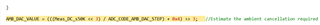The above line is used to calculate the ambient DAC code setting.

Let me explain with the ideal case. For example:

Let us assume PD current = 4uA

RF gain = 50k

Meas_DC_s50k = ((4e-6*2*50e3)*2^21/1.2) = 699050

AMB_DAC_VALUE = (((699050<<3)/87381)+4)>>3 = 8

For 4uA PD current, one will have set AMB DAC code of 8 to cancel the 4uA.

Thanks for the explanation. I'm still curious, so I'll ask you more.

(2*50e3*0.5e-6)*2^21/1.2

= 87381.33333333333333333333

• 50*e3 = 50k, 0.5e-6 = 0.5uA I know, but why multiply by 2?
• And *2^21/1.2 Can you tell where this part came from?
•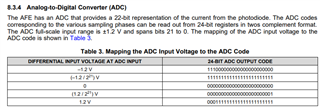• 8.3.4 Analog-to-Digital Converter (ADC) Do you mean this part? If so, can I see why you took reciprocal action?

Meas_DC_s50k = ((4e-6*2*50e3)*2^21/1.2) = 699050

• I couldn't find the expression in the code.
•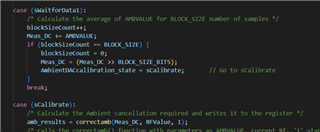• The code's all about collecting 8 AMBVALUE and shifting 3 times over.
• May I know where the formula is applied?

Thank you so much for replying to me every time.

Thank you

• Hi,

I will need to discuss with internal team and get back to you by next Monday.

Thanks

• Hi,

The factor 2 is due to datasheet page 14

"The signal current from the photodiode is converted to a differential voltage using a transimpedance amplifier (TIA)."..."The transimpedance gain between the input current and output differential voltage of the TIA is equal to 2 × Rf ."

Yes, 8.3.4 Analog-to-Digital Converter (ADC) and Table 3 show the conversion to convert ADC code to voltage after the TIA i.e. input to ADC.

dividied by 1.2 is to convert voltage to ADC value where the ADC's reference voltage is 1.2V

Thanks.

• Meas_DC_s50k = ((4e-6*2*50e3)*2^21/1.2) = 699050
• AMB_DAC_VALUE = (((699050<<3)/87381)+4)>>3 = 8

First, Meas_DC_s50k = (4e-6*2*50e3)*2^21/1.2) = 699050 I don't know where the formula is located in the calibration code.

In the code, simply add the AMBVALUE value, shift it, and multiply or divide the Rf_factor.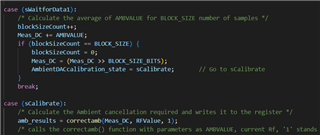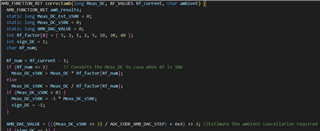Second, when calculating AMB_DAC_VALUE, divide it by ADC_CODE_AMB_DAC_STEP (65000), not 87381.

Why divide by 87381?.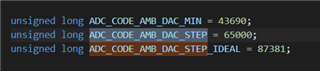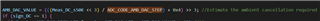Thank you.

• Hi,

Would you please clarify what you mean when you refer to "formula"?

Thanks

• Hi.

What you said

Meas_DC_s50k = ((4e-6*2*50e3)*2^21/1.2) = 699050

AMB_DAC_VALUE = (((699050<<3)/87381)+4)>>3 = 8

It means this calculation formula is not in the code.

Thank you.

• Hi,

AMB_DAC_VALUE = (((699050<<3)/87381)+4)>>3 = 8

are in the screenshot you attached earlier isn't it?

Thanks

• Hi,

AMB_DAC_VALUE = (((699050<<3)/87381)+4)>>3 = 8

are in the screenshot you attached earlier isn't it?

Thanks

• And, the Meas_DC_s50k = ((4e-6*2*50e3)*2^21/1.2) = 699050 is just an ideal case that we made up as an example for explanation based on some assumptions, not exactly implemented in the code.

"

Let me explain with the ideal case. For example:

Let us assume PD current = 4uA

RF gain = 50k

Meas_DC_s50k = ((4e-6*2*50e3)*2^21/1.2) = 699050

"

Thanks

• Hi.

If you look at the code, it's ADC_CODE_AMB_DAC_STEP = 65000, not ADC_CODE_AMB_DAC_STEP = 87381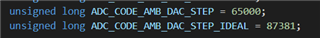Since the code uses ADC_CODE_AMB_DAC_STEP, AMB_DAC_VALUE=((699050<3)/87381)+4)>3=8 not AMB_DAC_VALUE=(((699050<3)/65000)+4)>3=8 is not correct?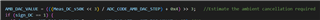Thank you.

• Hi,

87381 is IDEAL.

Please take a look of the reply earlier. it says&states at the very beginning, "IDEAL" and "20% accuracy".

You may use Ctrl+F  to search & find the two quoted words in this page and you shall see it.

Thanks